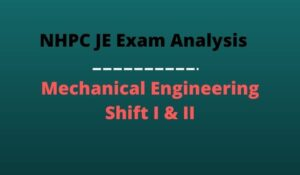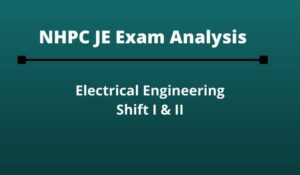Engineering Jobs   »   Civil Engineering quizs   »   NHPC-JE

# NHPC-JE’21 CE: Daily Practices Quiz. 01-Oct-2021

Know your strengths and practice your concepts with this quiz on NHPC JE Recruitment 2021. This quiz for NHPC JE Recruitment 2021 is designed specially according to NHPC Syllabus 2021.

Quiz: Civil Engineering
Exam: NHPC-JE
Topic: Miscellaneous

Each question carries 1 mark
Negative marking: 1/4 mark
Time: 8 Minutes

Q1. A stress element is subjected to tensile stress of 5 MPa on both the principal planes. The radius of Mohr Circle corresponding to this element will be
(a) 10 MPa
(b) 7.5 MPa
(c) 5 MPa
(d) Zero

Q2. Which of the following method is used to forecast the population of old and very large city?
(a) Arithmetical increase method
(b) Geometric progression method
(c) Graphical method
(d) Logistic curve method

Q3. Using Blasius equation, the friction factor for turbulent flow through pipes varies as:
(a) Re^(-1)
(b) Re^(-0.5)
(c) Re^(-0.33)
(d) Re^(-0.25)

Q4. If the Euler load for a column is 1000 kN and crushing load is 1200 kN, then the Rankine load will approximately be:
(a) 1200
(b) 600
(c) 900
(d) 545

Q5. Usually a canal is filling is provided with a side slope of (in case of loam soil)
(a) 1 : 1
(b) 1.25 : 1
(c) 1.5 : 1
(d) 2 : 1

Q6. The Los Angeles abrasion value (%) of aggregates to be used for the final layer of bituminous concrete is limited to:
(a) 25
(b) 30
(c) 40
(d) 50

Solutions

S1. Ans.(d)
Sol.σx = 5 MPa
σy = 5 MPa
Radius of Mohr circle = |(σx-σy)/2|
= |(5-5)/2|
= 0

S2. Ans.(a)
Sol. Arithmetical increase method is used to forecast the population of old and very large city. According to this method, the Population of town or city after ‘n’ decades is
▭(P_n=P_o+nx ̅ )
P_0= Present population
x ̅= Average of increase in population

S3. Ans.(d)
Sol. Friction factor according to Blasius equation,
f=0.316/(R_e^(1⁄4) )
f ∝1/(R_e^(1⁄4) )
f ∝ R_e^((-1)⁄4)
f ∝ R_e^(-0.25)

S4. Ans.(d)
Sol. Pe= 1000 KN
Pcr = 1200 KN
1/P=1/Pe+1/Pc
1/P=1/1000+1/1200
P= 545.45 KN

S5. Ans.(c)
Sol. The side slope of canal is provided in different condition as follows for loam soil
1. In cutting = H:V = 1:1
2. In filling = H:V = 1.5:1
And for sandy soil
1. In cutting = H:V = 1.5:1
2. In filling = H:V = 2:1

S6. Ans.(b)
Sol. The los angle abrasion value (%) of aggregate to be used for the final layer of bituminous concrete is limited to 30%.

Sharing is caring!

### TOPICS:

•NHPC Junior Engineer Syllabus 2023, Chec...
•NHPC JE Exam Pattern 2023, Latest Markin...
•NHPC JE Syllabus 2023, Download Latest S...
•NHPC Result Through GATE 2021, Download ...
•NHPC JE Mechanical Exam Analysis 2022 Di...
•NHPC JE Exam Analysis 2022 Electrical En...